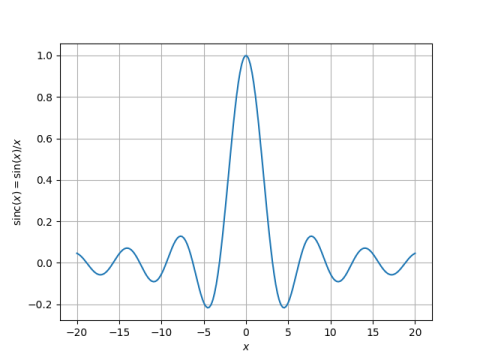# Reverse iteration root-finding

The sinc function is defined by

sinc(x) = sin(x)/x.

This function comes up constantly in signal processing. Here’s a plot.We would like to find the location of the function’s peaks. Let’s focus first on the first positive peak, the one that’s somewhere between 5 and 10. Once we can find that one, the rest will be easy.

If you take the derivative of sinc and set it to zero, you find that the peaks must satisfy

x cos x – sin x = 0

which means that

tan x = x.

So our task reduces to finding the fixed points of the tangent function. One way to find fixed points is simply to iterate the function. We pick a starting point near the peak we’re after, then take its tangent, then take the tangent of that, etc.

The peak appears to be located around 7.5, so we’ll use that as a starting point. Then iterates of tangent give

```     2.7060138667726910
-0.4653906051625444
-0.5021806478408769
-0.5491373258198057
-0.6119188887713993
-0.7017789436750164
-0.8453339618848119
-1.1276769374114777
-2.1070512803092996
1.6825094538261074
```

That didn’t work at all. That’s because tangent has derivative larger than 1, so it’s not a contraction mapping.

The iterates took us away from the root we were after. This brings up an idea: is there some way to iterate a negative number of times? Well, sorta. We can run our iteration backward.

tan x = x

we could equivalently solve

arctan x = x.

Since iterating tangent pushes points away, iterating arctan should bring them closer. In fact, the derivative of arctan is less than 1, so it is a contraction mapping, and we will get a fixed point.

Let’s start again with 7.5 and iterate arctan. This quickly converges to the peak we’re after.

```    7.721430101677809
7.725188823982156
7.725250798474231
7.725251819823800
7.725251836655669
7.725251836933059
7.725251836937630
7.725251836937706
7.725251836937707
7.725251836937707
```

There is a little complication: we have to iterate the right inverse tangent function. Since tangent is periodic, there are infinitely many values of x that have a particular tangent value. The arctan function in NumPy returns a value between -π/2 and π/2. So if we add 2π to this value, we get values in an interval including the peak we’re after.

Here’s how we can find all the peaks. The peak at 0 is obvious, and by symmetry we only need to find the positive peaks; the nth negative peak is just the negative of the nth positive peak.

The peaks of sin(x)/x are approximately at the same positions as sin(x), and so we use (2n + 1/2)π as our initial guess. In fact, all our peaks will be a little to the left of the corresponding peak in the sine function because dividing by x pulls the peak to the left. The larger x is, the less it pulls the root over.

The following Python code will find the nth positive peak of the sinc function.

```    def iterate(n, num_iterates = 6):
x = (2*n + 0.5)*np.pi
for _ in range(num_iterates):
x = np.arctan(x) + 2*n*np.pi
return x
```

My next post will revisit the problem in this post using Newton’s method.